## 1 Introduction

This paper extends the work of understanding the spectra X(n) begun in . These spectra were introduced by Ravenel in  and were used in an essential way in Devinatz, Hopkins and Smith’s proof of Ravenel’s Nilpotence Conjecture . These spectra are similar to the complex cobordism spectrum MU in a number of ways. Perhaps most importantly, much of the theory of complex orientations for ring spectra can be developed for X(n) by replacing $$\mathbb {C}P^\infty$$ with $$\mathbb {C}P^{n-1}$$ and formal group laws with formal group lawn-buds (cf. [9, Ch. II]). The interested reader can find a thorough description of the theory of complex orientations, both for MU and X(n), in [15, Sects. 4.1, 6.5]. The work contained in this paper, along with , also represents a small step in understanding chromatic homotopy theory and its fundamental objects, e.g. $$\mathbb {S}$$, MU and X(n), via the derived algebraic geometry framework developed in .

The main technical development of this paper is Theorem 1 in Sect. 2:

### Theorem

Let A be a connective $$\mathbb {E}_{k+1}$$-ring spectrum and choose an element $$\alpha \in \pi _d(A)$$ for $$d\ge 0$$ with associated self-map $${\bar{\alpha }}:\Sigma ^dA\rightarrow A$$. Then there is a map of A-modules $$cof({\bar{\alpha }})\rightarrow A/\!\! /_{\mathbb {E}_k}\alpha$$ which induces an isomorphism on homotopy groups in degrees less than 2d.

Here, the spectrum $$A/\!\! /_{\mathbb {E}_k}\alpha$$ is the versal$$\mathbb {E}_k$$-algebra onAof characteristic$$\alpha$$, described for $$k=\infty$$ in  and for all k in . It is constructed as by “attaching a d-cell” to A along $$\alpha$$ in the category of $$\mathbb {E}_k-A$$-algebras. Often, versal algebras of this form are conceptually interesting but have difficult to determine homotopy groups, so the above theorem may be of interest beyond its uses in this paper.

In Sect. 3 we apply the above theorem to prove that $$\pi _{2n-1}(X(n))$$ is cyclic, and that, moreover, it is generated by a certain element $$\chi _n$$ which was identified in . This is Corollary 2:

### Corollary

The element $$\chi _n\in \pi _{2n-1}(X(n))$$ generates $$\pi _{2n-1}(X(n))$$ for all $$n\ge 1$$.

These elements $$\chi _n$$ arise from the facts that X(n) is the Thom spectrum of a map $$\Omega SU(n)\rightarrow BGL_1(\mathbb {S})$$ and that $$\Omega SU(n)$$ sits in a fiber sequence $$\Omega SU(n)\rightarrow \Omega SU(n+1)\rightarrow \Omega S^{2n-1}$$. In  it was shown that X(n) is a quotient of $$\mathbb {S}$$ by an action of $$\Omega ^2 SU(n)$$. Because the action of $$\Omega ^2 SU(n)$$ on $$\mathbb {S}$$ factors through $$\Omega ^2 SU(n+1)$$, there is a residual action of $$\Omega ^2 SU(n+1)/\Omega ^2 SU(n)\simeq \Omega ^2 S^{2n-1}$$ on $$\mathbb {S}/\Omega ^2 SU(n)\simeq X(n)$$. This yields an $$\mathbb {E}_1$$-map $$\Omega S^{2n-1}\rightarrow BGL_1(\mathbb {S}/\Omega ^2 SU(n-1))\simeq BGL_1(X(n))$$. This is equivalent data to a map of pointed spaces $$S^{2n-2}\rightarrow BGL_1(X(n))$$, and we take $$\chi _n$$ to be the element canonically associated to it by [3, Definition 4.8].

It should be remarked that very little is known about the homotopy groups of X(n) in general. A complete knowledge of their homotopy groups is at least as difficult to obtain as complete knowledge of the stable homotopy groups of spheres. However, some computational progress has been made by Ravenel and others, and the state of the art can be found in .

While the above allows us to identify $$\chi _n$$ as a generator of $$\pi _{2n-1}(X(n))$$, and $$X(n+1)$$ as the versal $$\mathbb {E}_1-X(n)$$-algebra of characteristic $$\chi _n$$, this still relies on the somewhat geometric construction of $$\chi _n$$ given in . However, after localizing at a prime p, we see in Theorem 2 that simply attaching an $$\mathbb {E}_1$$-cell to X(n) along any generator of $$\pi _{2n-1}$$ will still produce (the p-localization of) $$X(n+1)$$:

### Theorem

The spectrum $$X(n+1)_{(p)}$$ can be obtained from $$X(n)_{(p)}$$ by attaching an $$\mathbb {E}_1-X(n)_{(p)}$$-cell along any generator of the cyclic group $$\pi _{2n-1}(X(n)_{(p)})$$.

We make special note of this only because of its similarity to the work of Priddy on constructing the Brown-Peterson spectrum BP, which is a summand of $$MU_{(p)}$$, by coning off all elements in odd degrees . The above theorem suggests that $$X(n+1)_{(p)}$$ is the spectrum obtained when one cones off the homotopy of $$X(n)_{(p)}$$ in degree $$2n-1$$ using an $$\mathbb {E}_1$$-cell (and that, in some poorly defined sense, $$MU_{(p)}$$ is obtained by coning off all homotopy in odd degrees, but with $$\mathbb {E}_1$$-cells). For the time being, this similarity appears to be entirely superficial. We still include the theorem however as it, at the very least, hints at a purely algebraic construction of MU, as requested by Ravenel in [15, Sect. 1.3].

Finally, in Sect. 4 we show that for any odd integer k there is a sequence of $$\mathbb {E}_k$$-ring spectra $$\{X(n,k)\}_{n\ge 0}$$ with $$X(0,k)\simeq \mathbb {S}$$ whose colimit over n is equivalent to MU. The spectrum X(nk) is the Thom spectrum of the base point component of the map $$\Omega ^k SU(n)\hookrightarrow \Omega ^k SU\simeq \mathbb {Z}\times BU$$. We prove that these spectra satisfy similar properties to the X(n) spectra. In particular we have Lemma 1 which completely describes their homotopy in low degrees

### Lemma

For odd k, the map $$X(n,k)\rightarrow MU$$ induced by the inclusion $$\Omega ^k SU(n)\hookrightarrow BU$$ induces an isomorphism on homotopy in degrees less than or equal to $$2n-k-1$$.

which generalizes the analogous statement for $$X(n,1)=X(n)$$ in [15, Sect. 6.5]. We also formulate suitably adjusted versions of Corollary 2 and Theorem 2 for the spectra X(nk).

These spectra may prove useful in understanding chromatic homotopy theory and the nilpotence detecting properties of MU, but because of the relative complexity of even their homology groups (as they are isomorphic to those of $$\Omega ^k SU(n))$$, we postpone a thorough investigation to future work.

There is also an “Appendix”, in which we prove several statements of a more category theoretic nature. These statements will be well known to experts. However, rigorous proofs of them in the language of quasicategories do not seem to be available in the literature, so we include them.

### 1.1 Notation and terminology

All of what follows uses the quasicategorical and derived algebra machinery of Lurie, as described in  and . Thus all of our categories will be quasicategories and any time we refer to a limit or colimit we will mean the quasicategorical limit or colimit as defined in . However, understanding the main statements in this paper should not require much specialized knowledge of Lurie’s work. We work specifically in the quasicategories of pointed Kan complexes (which we will refer to as spaces) and spectra. For both we will denote the tensor product by $$\wedge$$. We also use the shorthands $$[n]=\{0,1,\ldots ,n\}$$ and $$\langle n\rangle =\{*,1,\ldots ,n\}$$, where the latter is thought of as a pointed set. It seems unlikely that the theory of quasicategories is necessary to proving the results of this note. However, we make heavy use of results from  and , both of which use that language.

Most notation is described as it used in the text, but we will set a few standards here. By saying that a space or spectrum is n-connective, we mean that it has trivial homotopy groups below degreen. This should be contrasted with saying that an object is n-connected, which means that it has trivial homotopy groups in degrees less than or equal ton. For example, any space X is 0-connective, but is only 0-connected if $$\pi _0(X)\cong 0$$, and would be called 2-connective if it were “simply connected” in the classical sense. When a spectrum is 0-connective, we will just say that it is connective. A morphism of spaces or spectra will be called n-connective if it induces an isomorphism of homotopy groups in degress less than n.

We work throughout with the littlek-disks operads$$\mathbb {E}_k$$ for $$0\le k\le \infty$$ and freely use the fact that $$\mathbb {E}_1\simeq \mathbb {A}_\infty$$. We also remind the reader that an $$\mathbb {E}_0$$-algebra in a monoidal quasicategory is nothing more than an object equipped with a map from the monoidal unit. Given an $$\mathbb {E}_{k+1}$$-ring spectrum R, there is an $$\mathbb {E}_{k}$$-monoidal quasicategory of modules over R which we denote $$LMod_{R}$$. There is also a quasicategory of $$\mathbb {E}_k$$-algebras in $$LMod_{R}$$ which we denote by $$Alg_{R}^{\mathbb {E}_k}$$. Given an $$\mathbb {E}_k$$-ring spectrum R and $$0\le j<k$$, there always exists an $$\mathbb {E}_j$$-ring spectrum which is equivalent to R as a spectrum. When we wish to talk about this spectrum, we will simply say “R thought of as an $$\mathbb {E}_j$$-ring spectrum,” or “...as an $$\mathbb {E}_j$$-algebra.” The above construction induces a forgetful functor $$U_{\mathbb {E}_k}^{\mathbb {E}_j}:Alg_{\mathbb {S}}^{\mathbb {E}_k}\rightarrow Alg_{\mathbb {S}}^{\mathbb {E}_j}$$. This functor preserves limits and so has a colimit preserving left adjoint which we will denote by $$F_{\mathbb {E}_j}^{\mathbb {E}_k}$$. Given a fixed $$\mathbb {E}_k$$-ring spectrum R, an $$\mathbb {E}_j-R$$-algebra A for some $$0<j<k$$, and an integer $$0<l<j$$, we will denote the “free $$\mathbb {E}_l-A$$-algebra on an A-module M” by $$F^{\mathbb {E}_l}(M)$$, where the empty subscript indicates that M has no operadic structure. This also applies to the case $$k=j=\infty$$.

## 2 The effect of attaching a structured cell

In what follows, given an element $$\alpha \in \pi _d(A)$$ for an $$\mathbb {E}_{k+1}$$-ring spectrum A, the phrase attach an$$\mathbb {E}_k-A$$-cell along$$\alpha$$ means to produce the $$\mathbb {E}_k-A$$-algebra $$A/\!\! /_{\mathbb {E}_k}\alpha$$ defined by the following pushout in $$\mathbb {E}_k-A$$-algebras: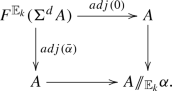In the above diagram, $${\bar{\alpha }}$$ is the morphism $$\Sigma ^dA\rightarrow A\wedge A\rightarrow A$$ induced by $$\alpha :\mathbb {S}^d\rightarrow A$$ and $$adj(-)$$ is the adjunction equivalence $$LMod_A(\Sigma ^d A,A)\overset{\sim }{\rightarrow }Alg_A^{\mathbb {E}_k}(F^{\mathbb {E}_k}(\Sigma ^d A), A)$$. The $$\mathbb {E}_k-A$$-algebra $$A/\!\! /_{\mathbb {E}_k}\alpha$$ is called the “versal $$\mathbb {E}_k-A$$-algebra of characteristic $$\alpha$$” in  because it always admits a not-necessarily-unique map to any other $$\mathbb {E}_k-A$$-algebra on which multiplication by $$\alpha$$ is nullhomotopic.

Our goal is to prove a lemma about elements in homotopy that always survive attaching $$\mathbb {E}_k$$-cells. Intuitively what we will prove is that, given a connective $$\mathbb {E}_{k+1}$$-ring spectrum A and $$\alpha \in \pi _d(A)$$ with induced self-map $${\bar{\alpha }}:\Sigma ^dA\rightarrow A$$, the spectrum $$A/\!\! /_{\mathbb {E}_k}\alpha$$ has a filtration whose associated graded A-module is $$cof({\bar{\alpha }})\oplus D$$ where D is 2d-connective. In particular, there is a map $$cof({\bar{\alpha }})\rightarrow A/\!\! /_{\mathbb {E}_k}\alpha$$ which is an isomorphism in $$\pi _i$$ for $$i<2d$$.

The main ideas behind the proof of the following Proposition 1 were explained to me by Tyler Lawson. We remind the reader that an $$\mathbb {E}_0-A$$-algebra is an A-module M equipped with a unit map $$A\rightarrow M$$. We also point out that we use $$\oplus$$ rather than $$\coprod$$ to denote the coproduct in the category $$LMod_A$$.

### Proposition 1

Let A be an $$\mathbb {E}_{k+1}$$-ring spectrum and $$F_{\mathbb {E}_0}^{\mathbb {E}_k}(M)$$ be the free $$\mathbb {E}_k$$-algebra on an $$\mathbb {E}_0-A$$-algebra M with unit map $$u:A\rightarrow M$$. If u induces an isomorphism on homotopy in degrees less than or equal to d, then the universal map $$\eta :M\rightarrow F_{\mathbb {E}_0}^{\mathbb {E}_k}(M)$$ induced by the free forgetful adjunction is and isomorphism on homotopy in degrees less than or equal to 2d.

### Proof

Let $$F^{\mathbb {E}_k}(M)$$ be the free $$\mathbb {E}_k$$-algebra on the R-module M (without its $$\mathbb {E}_0$$-structure). Note that $$F^{\mathbb {E}_k}(M)\simeq F_{\mathbb {E}_0}^{\mathbb {E}_k}(A\oplus M)$$ since $$A\oplus M$$ is the free $$\mathbb {E}_0$$-algebra on M and $$F^{\mathbb {E}_k}\circ F^{\mathbb {E}_0}\simeq F^{\mathbb {E}_k}$$. Thus we have an equivalence (of underlying A-modules) $$F_{\mathbb {E}_0}^{\mathbb {E}_k}(A\oplus M)\simeq \bigoplus _{n\ge 0}Sym_{\mathbb {E}_k}^n(M)$$. As such, by passing along this equivalence, there is an exhaustive filtration of $$F_{\mathbb {E}_0}^{\mathbb {E}_k}(A\oplus M)$$ whose filtration quotients are equivalent to $$Sym_{\mathbb {E}_k}^n(M)$$. In other words, the associated graded of this filtration is equivalent to $$F^{\mathbb {E}_k}(M)$$. Notice also that, since $$\oplus$$ is the biproduct in $$LMod_A$$, a morphism $$A\oplus X\rightarrow A\oplus Y$$ is entirely determined by a pair of maps $$X\rightarrow A$$ and $$X\rightarrow Y$$, so the induced map $$\bigoplus _{n\ge 0}Sym_{\mathbb {E}_k}^n(X)\rightarrow \bigoplus _{n\ge 0}Sym_{\mathbb {E}_k}^n(Y)$$ must respect the filtration induced by the grading. Thus we have filtration preserving morphisms $$F^{\mathbb {E}_k}(X)\rightarrow F^{\mathbb {E}_k}(Y)$$ whenever we have maps $$A\oplus X\rightarrow A\oplus Y$$.

Noticing that the pushout of the diagram in $$\mathbb {E}_0-A$$-algebras (which can be computed in A-modules) $$A\overset{1_A}{\leftarrow }A\overset{u}{\rightarrow }M$$ must be equivalent to M, we can use Lemma 2 to write M as the colimit of the simplicial object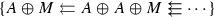with $$n^{th}$$ term equivalent to $$A\oplus A^{\oplus n}\oplus M$$. As a left adjoint, the functor $$F_{\mathbb {E}_0}^{\mathbb {E}_k}$$ commutes with colimits (where we are implicitly using that colimits of $$\mathbb {E}_0$$-algebras are computed in $$LMod_A$$), so we may write:Now, applying our filtration levelwise and using the fact that the induced maps respect filtration, we have a filtered simplicial object with filtration quotients: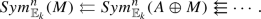Since each functor $$Sym^n_{\mathbb {E}_k}$$ preserves sifted colimits (of which simplicial resolutions are an example) by Lemma 4, we have that the filtration quotients of the induced filtration on $$F_{\mathbb {E}_0}^{\mathbb {E}_k}(M)$$ are equivalent to $$Sym_{\mathbb {E}_k}^n(cof(u))$$, i.e. the associated graded of the induced filtration on $$F_{\mathbb {E}_0}^{\mathbb {E}_k}(M)$$ is $$\bigoplus _{n\ge 0} Sym_{\mathbb {E}_k}^n(cof(u))$$. Here we are using the fact that the colimit of the simplicial object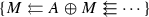is equivalent to (again by Lemma 2) the pushout of the diagram $$0\overset{0}{\leftarrow }A\overset{u}{\rightarrow }M$$, i.e. the cofiber of u.

Examining the filtration on this simplicial object more closely, and noticing that it arises from the “symmetric powers” filtration of $$F^{\mathbb {E}_k}(A\oplus A^{\oplus n}\oplus M)$$ at each simplicial degree, we see that its first two levels are the constant simplicial object: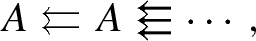and the constant simplicial object summed with the simplicial object we had before applying $$F_{\mathbb {E}_0}^{\mathbb {E}_k}$$, which has the form: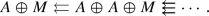These two objects have colimit A and M respectively. Thus we know that our filtration of $$F_{\mathbb {E}_0}^{\mathbb {E}_k}(M)$$ begins as $$A\overset{u}{\rightarrow }M$$. We know that the map from A to M is the unit map u because $$F_{\mathbb {E}_0}^{\mathbb {E}_k}(M)$$ must have the same unit as M (this also corresponds to the fact that the first filtration quotient must be equivalent to cof(u)). It is also clear that the filtration structure map $$M\rightarrow F_{\mathbb {E}_0}^{\mathbb {E}_k}(M)$$ must be the monadic unit map $$\eta$$. Hence the cofiber of the unit $$\eta :M\rightarrow F_{\mathbb {E}_0}^{\mathbb {E}_k}(M)$$ has a filtration with associated graded $$\bigoplus _{n\ge 2} Sym_{\mathbb {E}_k}^n(cof(u))$$. Thus the connectivity estimates of Lemma 3 give the result. $$\square$$

### Remark 1

There is nothing particular to the category $$LMod_A$$ that is necessary for the above constructions other than being a stable, cocomplete quasicategory and having a suitable notion of n-connective objects (i.e. a t-structure). Thus the above is a relatively general description of the functor $$F_{\mathbb {E}_0}^{\mathbb {E}_k}$$ for any $$k\ge 0$$.

### Theorem 1

Let A be a connective $$\mathbb {E}_{k+1}$$-ring spectrum and choose an element $$\alpha \in \pi _d(A)$$ for $$d\ge 0$$. Then there is a map of A-modules $$cof({\bar{\alpha }})\rightarrow A/\!\! /_{\mathbb {E}_k}\alpha$$ which is 2d-connective.

### Proof

By [3, Lemma 4.4] we have that $$A/\!\! /_{\mathbb {E}_k}\alpha \simeq F_{\mathbb {E}_0}^{\mathbb {E}_k}(cof({\bar{\alpha }}))$$. Since $$cof({\bar{\alpha }})$$ is the cofiber of a map $${\bar{\alpha }}:\Sigma ^dA\rightarrow A$$ which is d-connective, the unit map $$A\rightarrow cof({\bar{\alpha }})$$ is also d-connective. The result then follows from Proposition 1. $$\square$$

### Corollary 1

If A is an $$\mathbb {E}_{k+1}$$-ring spectrum with $$\alpha \in \pi _d(A)$$ and $$\pi _{d}(A/\!\! /_{\mathbb {E}_k}\alpha )\cong 0$$ then $$\alpha$$ generates $$\pi _d(A)$$ as a $$\pi _0(A)$$-module.

### Proof

By Theorem 1 there is a 2d-connective map of A-modules $$cof({\bar{\alpha }})\rightarrow A/\!\! /_{\mathbb {E}_k}\alpha$$. By considering the long exact sequence in homotopy for the cofiber sequence $$\Sigma ^dA\overset{{\bar{\alpha }}}{\rightarrow }A\rightarrow cof({\bar{\alpha }})$$, we see that we have an exact sequence of $$\pi _0(A)$$-modules

\begin{aligned} \pi _d(\Sigma ^dA)\cong \pi _0(A)\overset{-\cdot \alpha }{\rightarrow }\pi _d(A)\rightarrow \pi _d(cof({\bar{\alpha }}))\rightarrow \pi _{d-1}(\Sigma ^dA)\cong 0. \end{aligned}

From this it follows that $$\pi _d(A/\!\! /_{\mathbb {E}_k}\alpha )\cong \pi _d(cof({\bar{\alpha }}))\cong \pi _d(A)/[\alpha ]\pi _0(A)$$. Thus if $$\pi _d(A/\!\! /_{\mathbb {E}_k}\alpha )\cong 0$$ it must be the case that $$\pi _d(A)$$ is generated by $$[\alpha ]$$ as a $$\pi _0(A)$$-module. $$\square$$

## 3 $$\pi _{2n-1}(X(n))$$ and the construction of X(n) by attaching a cell

Corollary 1 immediately applies to $$\pi _{2n-1}$$ of the spectra X(n):

### Corollary 2

The element $$\chi _n\in \pi _{2n-1}(X(n))$$, from [5, Corollary 13], generates $$\pi _{2n-1}(X(n))$$ for all $$n\ge 1$$.

### Proof

From [5, Corollary 13] we know that $$X(n+1)\simeq X(n)/\!\! /_{\mathbb {E}_1}\chi _n$$. From the paragraph following Proposition 6.5.4 of  (or by noticing that $$X(n+1)$$ is the Thom spectrum of $$\Omega SU(n+1)\rightarrow BU$$, which is an equivalence below degree $$2n+1$$), we have that $$\pi _{2n-1}(X(n+1))\cong \pi _{2n-1}(MU)=0$$. Hence, by Corollary 1, $$\chi _n$$ must generate $$\pi _{2n-1}(X(n))$$. $$\square$$

Furthermore, if we localize at a prime, we need not have known about the elements $$\chi _n$$ at all. In other words, we can attach an $$\mathbb {E}_1$$-cell along any generator of $$\pi _{2n-1}$$ and still produce (the p-localization of) $$X(n+1)$$.

### Theorem 2

The spectrum $$X(n+1)_{(p)}$$ can be obtained from $$X(n)_{(p)}$$ by attaching an $$\mathbb {E}_1-X(n)_{(p)}$$-cell along any generator of the cyclic group $$\pi _{2n-1}(X(n)_{(p)})$$.

### Proof

Let $$\chi _{n,p}$$ be the image of $$\chi _n$$ under the p-localization map $$\pi _{2n-1}(X(n))\rightarrow \pi _{2n-1}(X(n)_{(p)})\cong \pi _{2n-1}(X(n))_{(p)}$$. From [12, Proposition 2.2.1.9] we know that the p-localization functor is symmetric monoidal, so preserves $$\mathbb {E}_n$$-algebras for all n. Additionally, from [12, Remark 7.3.2.13], and the results referenced in that remark, we know that this localization functor lifts to a left adjoint on categories of $$\mathbb {E}_n$$-algebras, so in particular preserves colimits of $$\mathbb {E}_1$$-algebras (for the analogous theorem in model categories, see [4, Theorem 5.6]). Thus we know that cell attachment commutes with localization, so $$X(n+1)_{(p)}$$ is equivalent to $$X(n)_{(p)}$$ with an $$\mathbb {E}_1-X(n)_{(p)}$$-cell attached along $$\chi _{n,p}$$.

Recall from [3, Lemma 4.4] that the versal $$\mathbb {E}_n$$-algebra on a class $$\alpha$$ is equivalent to the free $$\mathbb {E}_n$$-algebra on the $$\mathbb {E}_0$$-algebra $$cof({\bar{\alpha }})$$. This implies that $$X(n+1)\simeq F_{\mathbb {E}_0}^{\mathbb {E}_1}(cof({\bar{\chi }}_n))$$. Since localization, as a left adjoint, commutes with free functors, and preserves algebraic structure as described above, we can describe $$X(n+1)_{(p)}$$ as the free $$\mathbb {E}_1-X(n)_{(p)}$$-algebra induced up from the $$\mathbb {E}_0-X(n)_{(p)}$$-algebra $$cof({\bar{\chi }}_{n,p})$$.

We now have that $$\chi _{n,p}$$ generates $$\pi _{2n-1}(X(n)_{(p)})$$ as a finite module over the local ring $$\pi _0(X(n)_{(p)})\cong \mathbb {Z}_{(p)}$$. Any finite cyclic $$\mathbb {Z}_{(p)}$$-module is isomorphic to $$\mathbb {Z}/p^e$$ for some e, and an element in $$\mathbb {Z}_{(p)}$$ represents a generator if and only if it is not divisible by p. But these are precisely the units of $$\mathbb {Z}_{(p)}$$, so any other generator must differ from $$\chi _{n,p}$$ by a unit of $$\mathbb {Z}_{(p)}$$. If $$\alpha$$ is another generator, call the associated unit $$u_\alpha \in \pi _{0}(X(n)_{(p)})\cong \mathbb {Z}_{(p)}$$.

We have a commutative diagram whose rows are cofiber sequences:

The universal property of the cofiber induces a map $$\phi$$ filling in the diagram:

and an application of the 5-lemma in each degree of homotopy proves that $$\phi$$ is a homotopy equivalence. Notice also that the commutativity of the above diagram indicates that this is an equivalence of $$\mathbb {E}_0-X(n)$$-modules.

It follows then that the free $$\mathbb {E}_1$$-algebras on the equivalent $$\mathbb {E}_0$$-algebras $$cof({\bar{\alpha }})$$ and $$cof({\bar{\chi }}_{n,p})$$ are equivalent. Hence, as described above, [3, Lemma 4.4] implies that

\begin{aligned}X(n)_{(p)}/\!\! /_{\mathbb {E}_1}\alpha \simeq F_{\mathbb {E}_0}^{\mathbb {E}_1}(cof({\bar{\alpha }}))\simeq X(n+1)_{(p)}.\end{aligned}

$$\square$$

### Remark 2

We note that all of the above, and the results of , only succeed in producing $$X(n+1)$$ as an $$\mathbb {E}_1-X(n)$$-algebra. However, $$X(n+1)$$ is an $$\mathbb {E}_2-\mathbb {S}$$-algebra (though unpublished work of Lawson indicates that $$X(n+1)$$ is provably not $$\mathbb {E}_3$$), and this structure is not reflected in this work.

### Remark 3

Recalling that $$X(1)\simeq \mathbb {S}$$, $$\chi _1\simeq \eta$$ and $$cof(\eta )\simeq \Sigma ^{-2}\mathbb {C}P^2$$, we see that X(2) is equivalent to the free $$\mathbb {E}_1$$-ring spectrum on the $$\mathbb {E}_0$$-spectrum $$\Sigma ^{-2}\mathbb {C}P^2$$ (whose $$\mathbb {E}_0$$ structure is provided by the inclusion of the bottom cell $$\mathbb {S}\rightarrow \Sigma ^{-2}\mathbb {C}P^2$$). This should be compared to Hopkins’ description of $$X(2)_{(2)}$$ in .

### Remark 4

We have from  that $$H_*(X(n)_{(p)})\cong \mathbb {Z}_{(p)}[b_2,\ldots ,b_{2n-2}]$$ for all n. If there is no m such that $$n= p^m-1$$, $$X(n+1)_{(p)}$$ is equivalent to an infinite wedge of suspended copies of $$X(n)_{(p)}$$. In particular, one has a copy of $$X(n)_{(p)}$$ for each power of the polynomial generator $$b_n\in \pi _{2n-2}(X(n)_{(p)})$$  (this can also be deduced from [15, Chapter 6]). In other words, the fact that $$H_*(X(n+1)_{(p)})$$ is a polynomial algebra over $$H_*(X(n)_{(p)})$$ is manifested at the level of spectra whenever passing from n to $$n+1$$ does not increase the largest power of p such that $$p^m\le n$$.

This indicates that the p-localization of the element $$\chi _n$$, and hence the homotopy group it generates, is zero when n is not one less than a power of a p. Theorem 2 implies that for $$n=p^m-1$$ for some m, every other generator of $$\pi _{2n-1}(X(n))$$ is of the form $$r\chi _n$$ for an integer r which is not divisible by p. So in this case we can conclude that $$\pi _{2n-1}(X(n))$$ must have been $$\mathbb {Z}/p^e$$ for some e. We leave the detailed investigation of this structure, and these homotopy groups, to a future paper.

### Remark 5

From [16, Example 1.22], we can see that $$\chi _{p-1}$$ becomes (a sum of copies of) $$\alpha _1$$ when localized at p. It seems likely that the p-localization of $$\chi _{p^m-1}$$ recovers the $$m^{th}$$ Greek letter element of the p-localized stable homotopy groups of spheres.

## 4 $$\mathbb {E}_k$$-monoidal analogues of X(n)

We now introduce a new sequence of spectra which are $$\mathbb {E}_k$$-monoidal, have colimit equivalent to MU, and which are (eventually) produced by attaching multiplicative cells. We generalize Corollary 2 and Theorem 2 to these new spectra.

### Definition 1

For an odd integer k, let X(nk) be the sequence of spectra obtained by Thomifying (the zero components of) the sequence of $$k+1$$-fold loop spaces

\begin{aligned} *\simeq \Omega ^kSU(1)\rightarrow \Omega ^kSU(2)\rightarrow \cdots \rightarrow \Omega ^kSU(n)\rightarrow \cdots \rightarrow \Omega ^kSU\simeq \mathbb {Z}\times BU. \end{aligned}

### Remark 6

From [3, Theorem 2.8] we can deduce that the spectra X(nk) are $$\mathbb {E}_{k+1}$$-monoidal ring spectra. This is a classical result of Lewis , and is also shown specifically for Thom spectra in [1, Theorem 1.7].

### Theorem 3

For $$n\ge \frac{k-1}{2}$$ there is an element $$\chi _{n,k}\in \pi _{2n-k}(X(n,k))$$ such that the spectrum $$X(n+1,k)$$ is equivalent to X(nk) with an $$\mathbb {E}_k-X(n,k)$$-cell attached along $$\chi _{n,k}$$.

### Proof

Fix n and k and assume $$n\ge \frac{k-1}{2}$$. Recall that there is a fiber sequence $$SU(n)\rightarrow SU(n+1)\rightarrow S^{2n+1}$$. By applying the based loops functor k times we obtain a fiber sequence $$\Omega ^kSU(n)\rightarrow \Omega ^k SU(n+1)\rightarrow \Omega ^kS^{2n+1}$$. If $$2n+1\ge k$$ then the base space of the preceding fibration is equivalent to the free $$\mathbb {E}_k$$-algebra on $$S^{2n+1-k}$$. By [5, Theorem 1] there is a morphism of $$\mathbb {E}_{k}$$-spaces $$\phi :\Omega ^k\Sigma ^kS^{2n+1-k}\rightarrow BGL_1(X(n,k))$$ whose associated X(nk)-algebra Thom spectrum is equivalent to $$X(n+1,k)$$ as an $$\mathbb {E}_k-X(n,k)$$-algebra.

Since the domain of the map $$\phi$$ is the free $$\mathbb {E}_k$$-algebra on $$S^{2n+1-k}$$, we have, by the free-forgetful adjunction, a map of based spaces $$\phi ':S^{2n+1-k}\rightarrow BGL_1(X(n,k)).$$ By the loops-suspension adjunction, and the fact that $$GL_1(X(n,k))$$ is equivalent to $$\Omega BGL_1(X(n,k))$$, we thus have a map of based spaces $$\phi '':S^{2n-k}\rightarrow GL_1(X(n,k))$$. Note that, since $$GL_1(X(n,k))$$ is composed of the connected components of the units in $$\pi _0(\Omega ^\infty X(n,k))\cong \mathbb {Z}$$, the inclusion $$i:GL_1(X(n,k))\hookrightarrow \Omega ^\infty X(n,k)$$ is not based. In particular, the base point of $$GL_1(X(n,k))$$ corresponds to 1 and the base point of $$\Omega ^\infty X(n,k)$$ corresponds to 0. Let $$u=\pm 1$$ be the connected component containing the image of $$\phi ''$$. Then there is a translation map $$\tau _u:\Omega ^\infty X(n,k)\rightarrow \Omega ^\infty X(n,k)$$ such that the composition $$\tau _u\circ i\circ \phi ''$$ is a based map $$S^{2n-k}\rightarrow \Omega ^\infty X(n,k)$$. Finally, by the adjunction between $$\Omega ^\infty$$ and $$\Sigma ^\infty$$, we obtain a map of spectra $$\mathbb {S}^{2n-k}\rightarrow X(n,k)$$. This defines our element $$\chi _{n,k}\in \pi _{2n-k}(X(n,k))$$. An application of [3, Theorem 4.10] gives that $$X(n+1,k)$$, the Thom spectrum of the map $$\phi$$ that we began with, is equivalent to $$X(n,k)/\!\! /_{\mathbb {E}_k}\chi _{n,k}$$. $$\square$$

### Corollary 3

For $$n\ge \frac{k-1}{2}$$, there is a Thom isomorphism of $$\mathbb {E}_k-X(n,k)$$-algebras

\begin{aligned} X(n+1,k)\wedge _{X(n,k)}X(n+1,k)\simeq X(n+1,k)\wedge _\mathbb {S}\Omega ^k\Sigma ^k S^{2n+1-k}. \end{aligned}

### Proof

This is precisely the Thom isomorphism of [1, Theorem 8.4] used along with [3, Corollary 3.17]. In particular, since $$X(n+1,k)$$ is a Thom spectrum associated to a map of $$\mathbb {E}_k$$-spaces $$\Omega ^k\Sigma ^kS^{2n+1-k}\rightarrow BGL_1(X(n,k))$$ it is oriented with respect to itself, so admits a Thom isomorphism. $$\square$$

We emulate Corollary 2 and Theorem 2 below as Corollaries 4 and 5 after confirming a fact about X(nk) that was shown for $$X(n)=X(n,1)$$ in .

### Lemma 1

For odd k, the map $$X(n,k)\rightarrow MU$$ induced by the inclusion $$\Omega ^k SU(n)\hookrightarrow BU$$ is an isomorphism on homotopy groups in degrees less than or equal to $$2n-k-1$$.

### Proof

First recall that the inclusion $$SU(n)\hookrightarrow SU$$ is an equivalence in degrees less than 2n. It follows then that, after applying $$\Omega ^k$$, for odd k, $$\Omega ^k SU(n)\rightarrow BU$$ is an equivalence in degrees less than $$2n-k$$.

Now recall that the Thom spectrum functor (being computable as a colimit itself as in  or as a left adjoint as in ) preserves colimits. Thus the cofiber of the map $$Th(i):X(n,k)\rightarrow MU$$ is the Thom spectrum of the cofiber of $$i:\Omega ^k SU(n)\rightarrow BU$$ (taken in the category of spaces over BU). Note that cofibers in overcategories are computed in the underlying categories, so the homotopy groups of cof(i) as a space are isomorphic to the homotopy groups of cof(i) as a space over BU. Clearly the space cof(i) has trivial homotopy groups in degrees less than $$2n-k$$.

Consider the pointed map $$BU\rightarrow BGL_1(\mathbb {S})\rightarrow BGL_1(H\mathbb {Z})$$ given by the complex J-homomorphism followed by the delooping of the canonical map $$GL_1(\mathbb {S})\rightarrow GL_1(H\mathbb {Z})$$. This map is null because $$BGL_1(H\mathbb {Z})\simeq \mathbb {R}P^\infty$$ and $$H^1(BU;\mathbb {Z}/2)=0$$. So we have, via the orientation theory of , the following three equivalences: $$H\mathbb {Z}\wedge X(n,k)\simeq H\mathbb {Z}\wedge \Sigma ^\infty _+\Omega ^k SU(n)$$, $$H\mathbb {Z}\wedge MU\simeq H\mathbb {Z}\wedge \Sigma ^\infty _+BU$$ and $$H\mathbb {Z}\wedge Th(cof(i))\simeq H\mathbb {Z}\wedge \Sigma ^\infty _+ cof(i)$$. In other words, we have Thom isomorphisms in integral homology for all three Thom spectra. Since we know that cof(i) must have trivial homotopy groups in degrees less than $$2n-k$$, it also has trivial homology in those degrees. Hence Th(cof(i)) also has trivial homology in those degrees and thus trivial homotopy there as well (as it is connective). So the Thom spectrum functor preserves connectivity of the map $$\Omega ^kSU(n)\rightarrow BU$$, causing the map $$X(n,k)\rightarrow MU$$ to be an equivalence in degrees less than $$2n-k$$. $$\square$$

### Remark 7

It follows from the above, and the fact that $$\pi _*(MU)$$ is trivial in odd degrees, that $$\pi _{2n-k}(X(n+1,k))=0$$ because $$2n-k$$ is odd and $$2n-k<2(n+1)-k$$.

### Corollary 4

The elements $$\chi _{n,k}$$ described in Theorem 3 generate $$\pi _{2n-k}(X(n,k))$$ as abelian groups.

### Proof

Since $$X(n+1,k)\simeq X(n,k)/\!\! /_{\mathbb {E}_k}\chi _{n,k}$$, this follows immediately from Theorem 1 and Remark 7. We are also using the fact that $$\pi _0(X(n,k))\cong \mathbb {Z}$$. Note that this corollary is entirely analogous to Corollary 2. $$\square$$

### Corollary 5

If $$p\in \mathbb {Z}$$ is a prime and $$n\ge \frac{k-1}{2}$$ then $$X(n+1,k)_{(p)}$$ is equivalent to $$X(n,k)_{(p)}$$ with an $$\mathbb {E}_k$$-cell attached along any generator of $$\pi _{2n-k}(X(n,k)_{(p)}$$.

### Proof

The proof proceeds completely identically to the proof of Theorem 2. $$\square$$

### Remark 8

Recall that the spectra $$X(n,1)=X(n)$$ have an interpretation in terms of truncated formal group laws, or formal n-buds, as described in [15, Sect. 6.5]. The author is not aware of any similar interpretation of the spectra X(nk) for $$k>1$$. Such an interpretation, even for $$k=3$$ in light of the appearance of the space $$\Omega ^3 SU(n)$$ in the proof of the Nilpotence Conjecture, would likely be enlightening.